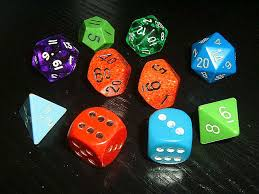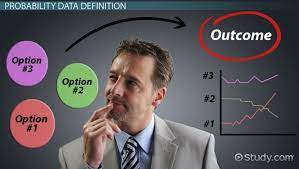Business decisions are being analyzed based on uncertainty, that is what is the likely sales will be affected if we increase prices, or the chances of increase in production if we purchase new equipment, or the odds of investing in a stock market will be profitable. All these are probability-driven, and that is why probability is the numeric measure of the likelihood an event will occur.

In making a decision for a business in the face of uncertainty, they are some parameters you must put into considerations. One of them is the experiment, this is the process of generating a well-defined outcome, let us refer to the probability we stated above. What is the likelihood that sales will be affected, if we increase the price, this the experiment, the second parameter is sample space, this is used to determine the experimental outcome, so for the example stated above, the experimental outcome will now be increased in sales or decrease in sales? The third parameter is the sample point, this is refer to any one particular experimental outcome, that is the increase in sales or decrease in sales.

In decision-making for business, one should consider that the notion of an experiment is somewhat different from the laboratory sciences. In the laboratory sciences, the researcher assumes that each time an experiment is repeated in exactly the same way, the same outcome will occur. For the type of experiment in probability, the outcome is determined by chance. Even though the experiment might be repeated in exactly the same way, a different outcome may occur. Because of this difference, the experiments we study in probability are sometimes called random experiments. A good example of this, consider a company is trying to introduce a new product to the market, decided to conduct research, the salesperson placed a spot, and the salesperson randomly markets the product to 400 people and 75 people bought the product. If the research is conducted again, making every other condition constant, there is a higher probability 75 people will not buy the product, it may be higher or lower.

Probability information and economic measures create the foundation for quantitative analysis. There are many ways that business decisions can be made. One type of decision-making analysis involves using probabilities and economic measures to make decisions. To help Mr. Adim make his decision, let’s see how this type of analysis works.

Mr. Adim is wondering how he should make her decision about opening a new plant. He thinks that crunching numbers will be better than just going with her gut or with what her company has always done, but there are so many possible outcomes: the new plant could make a ton of money, or it could tank and end up causing her company to go into bankruptcy. How can he figure out the best option?

One way to do that is to use the expected value of the different outcomes, which is the weighted payoff based on probabilities. For example, if Mr. Adim believes that there’s a 40% chance that the second plant will make a profit of \$100,000 in its first year, then the expected value of that plant is \$100,000 x .4, or \$40,000.

But, let’s say that there’s also a 30% chance that the second plant will only make \$10,000 in that first year. That expected value is \$3,000. And if there’s a 20% chance that the plant will lose \$100,000 in the first year, then the expected value is -\$20,000.

So, which option should Mr. Adim go with when trying to make her decision? One thing Mr. Adim can do is to add up the different options. \$40,000 + \$3,000 + -\$20,000 = \$23,000. That’s the total expected value of what might happen in the first year of the plant’s opening.

How does Mr. Adim figure out the probability of different outcomes? There are two ways to calculate the expected value. The first is to calculate it based on objective probability or use actual data to figure out the probability. For example, if Mr. Adim has data that shows that similar manufacturing plants made \$100,000 profit in their first year about 40% of the time, that’s objective probability; he’s using actual data to figure out the probability of her plant making \$100,000 profit in the first year.

But, what if he doesn’t have data to use? The other way to calculate the expected value is with subjective probability or using a rough estimate to figure out the probability.

By so doing Mr. Adim will be able to base his decisions on facts available, instead of speculations.## PROBABILITY – A TOOL FOR DECISION MAKING

in## “MAXIMIZING BALANCE SHEET: UNLOCKING THE PATH TO ORGANIC GROWTH AND FINANCIAL SUCCESS.

in